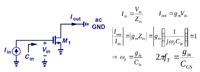Is the device capacitance bias-dependent or independent?

1. Is the device capacitance bias-dependent or independent?

Hi, I would like to know if the device intrinsic capacitance like Cgs bias-dependent or independent. Giving say, bias 400mV or 500mV (common source circuit with Vgs = 400mV or 500mV) for 65nm technology, is the Cgs the same or different and why？

Thanks,
Alex•

2. Re: Is the device capacitance bias-dependent or independent?

Yes the Cgs capacitance is voltage dependent capacitance.
Hope the following references will help:

1) Chapter 2, Section 2.4.2 Mos Device Capacitance Page No: 29 from the book http://www.ax-09.ru/gruppa/materials...20Circuits.pdf

2) The Voltage-dependent capacitors section from http://en.wikipedia.org/wiki/Capacitance

3) http://www.ee.iitm.ac.in/~nagendra/E...s/20060110.pdf

Hope it will help ... :)

1 members found this post helpful.3. Re: Is the device capacitance bias-dependent or independent?

Thanks a lot!By the way, in your third source, in Unity Gain frequency fT of MOS transistor part, do you know what does the (s) in Id(s) and 1/s in Vgs(s) mean?

- - - Updated - - -Originally Posted by Alex LiaoThanks a lot!By the way, in your third source, in Unity Gain frequency fT of MOS transistor part, do you know what does the (s) in Id(s) and 1/s in Vgs(s) mean?
Is s = 2 * pi * f?•

4. Re: Is the device capacitance bias-dependent or independent?

Anything with a depletion region on either side of it will be
voltage dependent. MOS channels swing from accumulation
through depletion to inversion. Cgs follows gate voltage
directly; Cgd, only when the channel is well lit near the drain
(linear region - when it goes constant current the capacitance
is sort of stood off from the channel by the pinch. The
partitioning with drain voltage is a messy thing to get right.

The overlap capacitances are less voltage dependent, if
you do not have poly depletion anyway. Mostly fringing
fields through the various oxides. You can't meaningfully
deplete a >1E20 doped S/D region even if it wasn't silicided.

1 members found this post helpful.5. Re: Is the device capacitance bias-dependent or independent?Originally Posted by Alex LiaoIs s = 2 * pi * f?
s is a complex variable in Laplace domain.

1 members found this post helpful.6. Re: Is the device capacitance bias-dependent or independent?

Hi Alex LiaoOriginally Posted by Alex LiaoThanks a lot!By the way, in your third source, in Unity Gain frequency fT of MOS transistor part, do you know what does the (s) in Id(s) and 1/s in Vgs(s) mean?
Agreeing with , Dominik Przyborowski, the s is the complex variable in Laplace domain. They are calculating the transfer function Id/Ig in Laplace domain for calculation of Transit frequency ft.
The transit or cut-off frequency, fT, is a measure of the intrinsic speed of a transistor, and is defined as the frequency where the current gain falls to 1.

Hope the picture explains:1 members found this post helpful.•

7. Re: Is the device capacitance bias-dependent or independent?

Thank you (SIDDHARTHA HAZRA, dick_freebird and Dominik Przyborowski) so much!--[[ ]]--